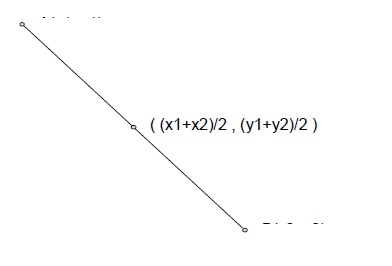# Program to find the mid-point of a line in C++

In this problem, we are given two points A and B, starting and ending point of a line. Our task is to create a program to find the mid-point of a line in C++.

Problem Description − Here, we have a line with starting and ending points A(x1, y1) and B(x2, y2). And we need to find the mid-point of the line.

Let’s take an example to understand the problem,

## Input

a(x1, y1) = (4, -5)
b(x2, y2) = (-2, 6)

## Output

(1, 0.5)

## Explanation

(x1 + x2)/2 = 4 - 2 / 2 = 1
(y1 + y2)/2 = -5 + 6 / 2 = 0.5

## Solution ApproachTo solve the problem, a simple method is using the geometrical formula for the mid of a line. The formula is given by,

Mid = ( ((x1 + x2)/2), ((y1 + y2)/2) )

Program to illustrate the working of our solution,

## Example

Live Demo

#include<iostream>
using namespace std;
int main() {
float point = {{-4, 5}, {-2, 6}};
float midX = (float)(( point + point)/2);
float midY = (float)(( point + point)/2);
cout<<"The mid-points are ("<<midX<<" , "<<midY<<")";
return 0;
}

## Output

The mid-points are (-3 , 5.5)